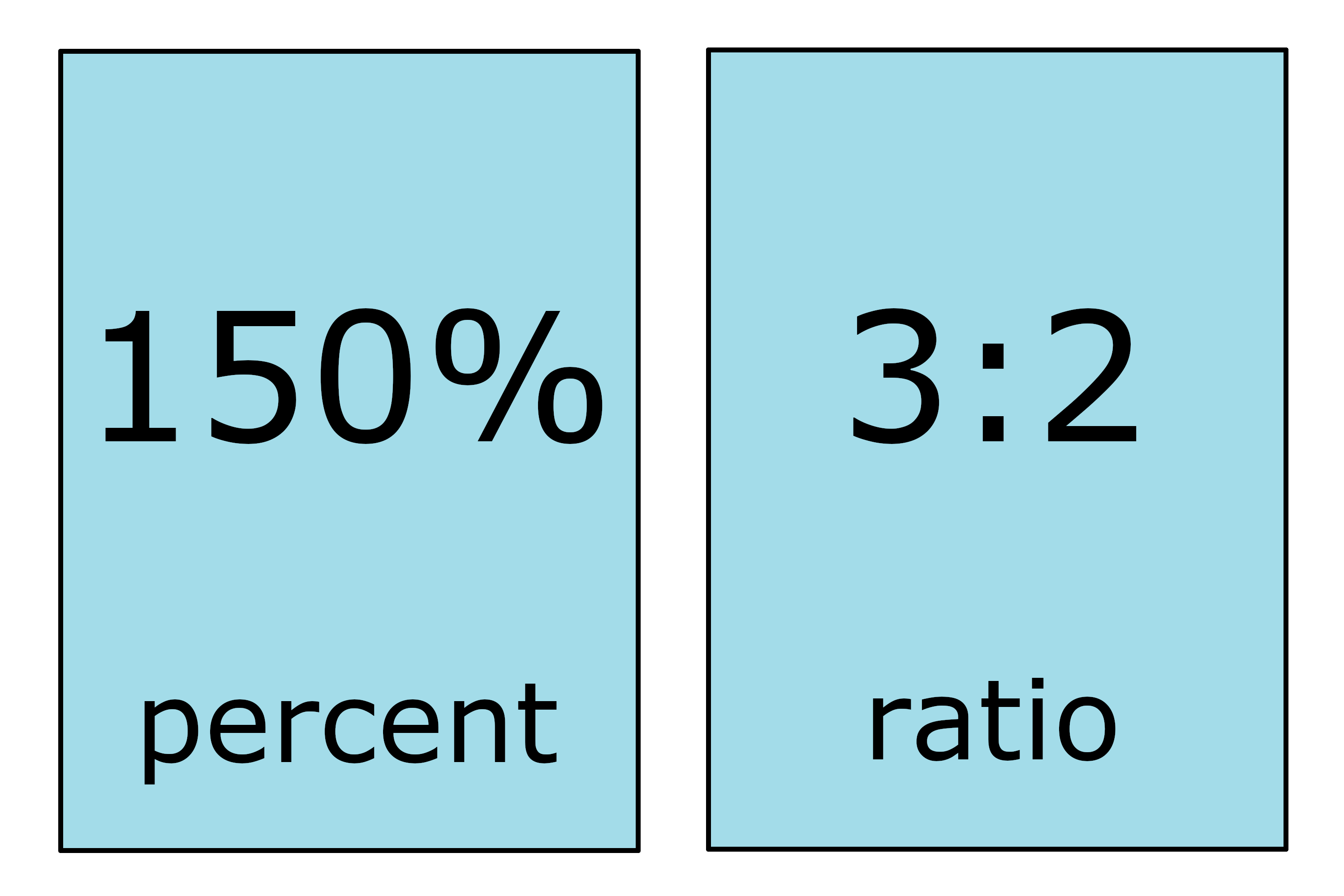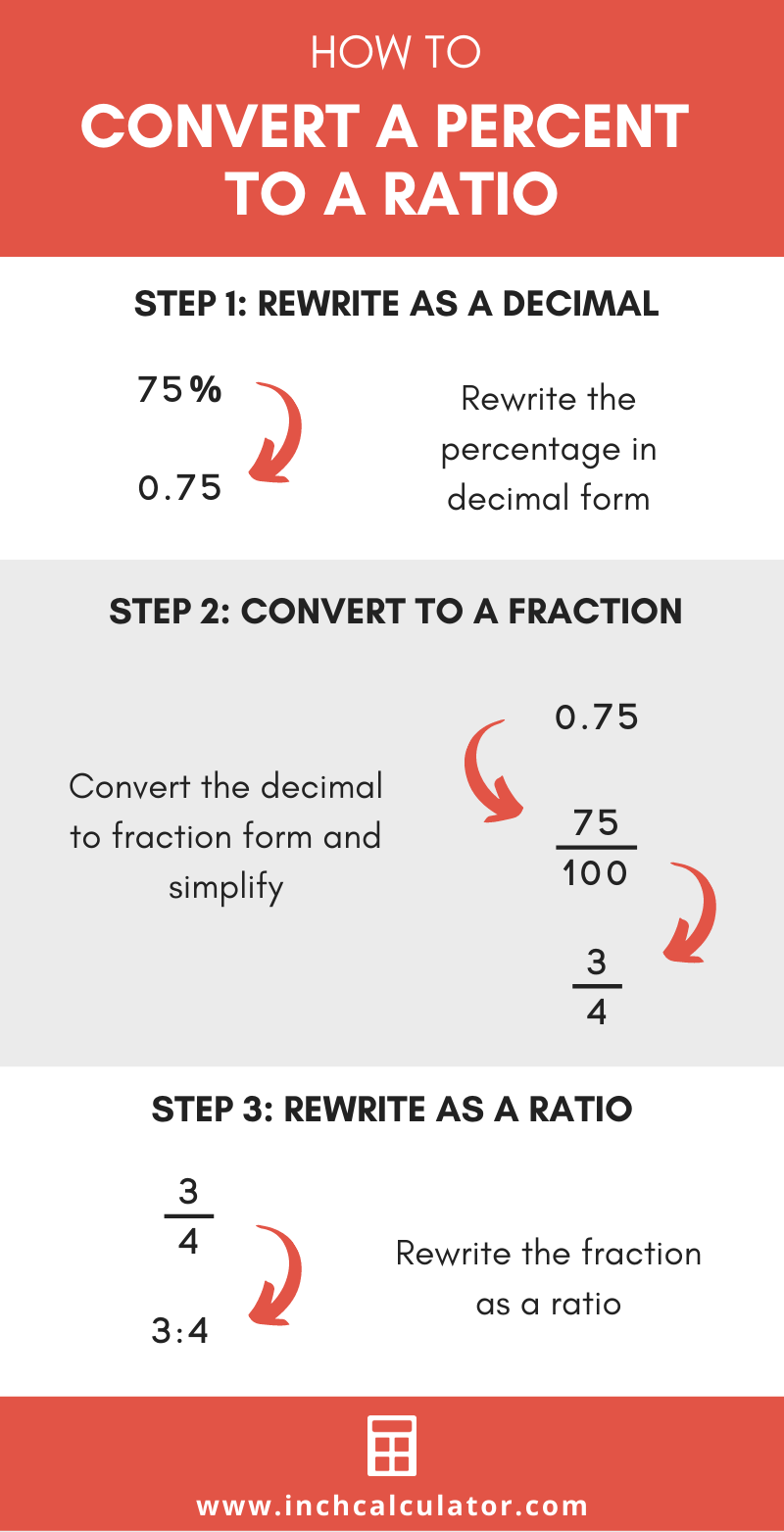# Percent to Ratio Calculator

Convert a percent to a ratio expressed as A:B by entering percentage below.

%

## Ratio:

3 : 4

### Steps to Convert a Percent to a Ratio

Step One: Convert the percentage to a decimal
75%=0.75
Step Two: Convert from decimal form to fraction
0.75=3 / 4
Step Three: Rewrite as a ratio
3 / 4=3:4
Learn how we calculated this below

## How to Convert a Percentage to Ratio Form

Percentage values are often expressed as a whole number with a percent symbol (%) after the number, while ratios are usually written using two numbers separated by a colon (:). You can convert a value expressed as a percent to a ratio in a few short steps.

### Step One: Rewrite as a Decimal

First, rewrite the percentage value as a decimal number. If your percentage is already in decimal form then, you can skip this step. To convert to a decimal, simply divide the percentage by 100 and remove the percent symbol.

### Step Two: Convert to a Fraction

Then, convert the decimal number to a fraction. This can be done by placing the decimal value over 1 to get the fraction. The decimal value will be the fraction numerator, and 2 will be the denominator.

If the decimal number, now the fraction numerator, is not a whole number, then multiply both the numerator and denominator by 10 until the numerator is a whole number. This will yield an equivalent fraction with no decimal points.

At this point, you can reduce the fraction as needed.

If you’re not feeling comfortable with this step, then use a decimal to fraction calculator for the conversion.

### Step Three: Rewrite as a Ratio

The final step is to rewrite the fraction from the previous step in ratio form. To do this, simply replace the fraction bar with a colon (:). Place the fraction numerator in front of the denominator and put a colon between them. Learn more about converting a fraction to a ratio.

For example, convert 150% to a ratio.

Convert to a decimal first.
150% = 150 ÷ 100
150% = 1.5

Convert to a fraction.
1.5 = 1.5 / 1
1.5 / 1 = (1.5 × 10) / (1 × 10)
1.5 / 1 = 15 / 10

Reduce the fraction.
15 / 10 = (15 ÷ 5) / (10 ÷ 5)
15 / 10 = 3 / 2

Rewrite as a ratio.
3 / 2 = 3:2You’ll probably also like our ratio to percentage calculator which shows you how to reverse this.